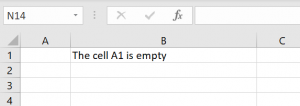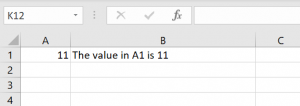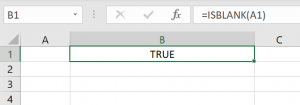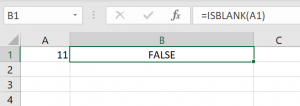### Isempty function in VBA

In this article, you will learn how to use the IsEmpty function in VBA to check if a cell is empty. If you want to do the same in Excel, a similar function is the IsBlank function. In this step-by-step tutorial, for all levels of Excel and VBA users, you will see how to use both functions.

## Using the IsEmpty function in VBA

As we already mentioned in the introduction, the IsEmpty is the simple function in VBA which checks if a cell is empty. If the selected does not contain any value, the function will return Boolean TRUE. On the other side, if the cell contains a value, the function returns FALSE. Here is the code:

In the example, we want to check if the cell A1 in the Sheet1 contains any value. Therefore, if the cell is empty, we will return “The cell A1 is empty” in the cell B1. If the cell contains a value, we will return the value of the cell A1 in the cell B1. Let’s run the code first with empty A1 and then with A1 containing some value:Image 1. Using the IsEmpty in VBA with the empty cellImage 2. Using the IsEmpty in VBA with populated cell

## Using the IsBlank function in Excel

The IsBlank function also checks if the value of the cell is blank, but this function does not exist in VBA. We’ll see on similar examples how to check if the cell is blank in Excel, using this formula:Image 3. Using the IsBlank in Excel with blank A1 cellImage 4. Using the IsBlank in Excel with populated A1 cell

As you can see, we check if the cell A1 is blank and return the result of the function in the cell B1. In Image 3, the result of the function is Boolean TRUE. On the other side, in Image 4, the result of the function is FALSE, as the cell A1 is “11” and is not blank.

#### You may also like some of this related content...

VBA has many string functions that will allow you to manipulate and work with text
VBA Round The VBA Round Function rounds numbers to a specified number of digits. Syntax
While working in VBA, you often need to convert strings into lowercase, uppercase or proper
Every character in Excel has an assigned number code to itself. The Chr function in
The following UDF will return the formulae for a given cell reference: VBA Function to